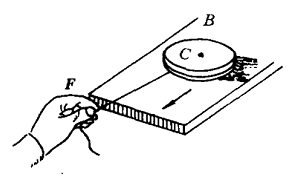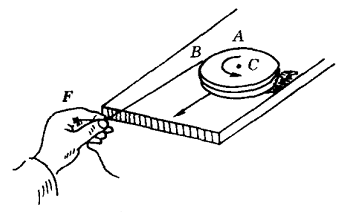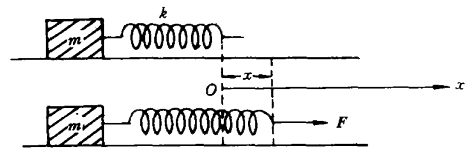# 关于功的定义的一点意见W＝mvC2/2s平动＝vCdt

s转动＝Rωdt

s＝s平动＋s转动＝vCdt＋Rωdt

$\begin{array}{*{20}{l}}{W = \int_0^t {F({v_C}dt + R\omega dt)} }\\{ = F\left[ {\int_0^t {{a_C}dt + R\int_0^t {{\beta _C}tdt} } } \right]}\\{ = F\left[ {\frac{1}{2}{a_C}{t^2} + R\frac{1}{2}{\beta _C}{t^2}} \right]}\\{ = \frac{1}{2}ma_C^2{t^2} + ma_C^2{t^2}}\\{ = \frac{3}{2}ma_C^2{t^2}}\\{ = \frac{3}{2}mv_C^2}\end{array}$

E平动＝mvC2/2＝maC2t2/2 （1）

E转动＝ ICωC2/2 （2）

$${{\rm{I}}_{\rm{C}}} = \frac{1}{2}{\rm{m}}{{\rm{R}}^{\rm{2}}}$$，I为圆盘对通过质心的垂直轴的转动惯量，ωC为圆盘在t时刻绕质心的转动角速度。因为：

ωC＝βCt

F＝maC

FR＝ICβC

βC是盘绕质心转动的角加速度。因为：

$${\beta _{\rm{C}}}{\rm{ = }}\frac{{FR}}{{{I_C}}} = \frac{{2{a_C}}}{R}$$

ωC＝2aCt/R

E转动＝maC2t2

E＝E平动＋E转动＝$$\frac{3}{2}{\rm{m}}{{\rm{a}}_{\rm{C}}}^{\rm{2}}{{\rm{t}}^{\rm{2}}} = \frac{3}{2}{\rm{m}}{{\rm{v}}_{\rm{C}}}^{\rm{2}}$$2006 - 2023，推荐分辨率1024*768以上，推荐浏览器Chrome、Edge等现代浏览器，截止2021年12月5日的访问次数：1872万9823 站长邮箱